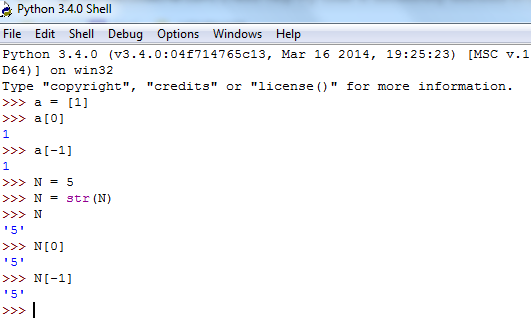# Need Help! my code is successfully executed in Code,Compile & Run Panel of Codechef but on submission shows Wrong answer. The Question Code is FLOW004 (first and last digit) of Begineer level.And, my code is:

``````
#include <iostream>
using namespace std;

int main() {
int t;
cin>>t;
if(t>=1 && t<=1000)
{
for(int i=0;i<t;i++)
{
long n;
long r=0;
int ld=0,fd=0;
cin>>n;
if(n>=1 && n<=1000000)
{

ld=n%10;
for(long j=0;j<n;j++)
{
r=n/10;
if(r<10)
{
fd=(int)r;
break;
}
}
}
cout<<fd+ld;
}
}
return 0;
}
``````
1 Like

This is not your responsibility, The input used during testing is provided within the constraints ,don’t worry. Also, for last digit, you are not changing n value at each iteration, so ld will remain 0 and your program gives wrong answer.

Also, the ‘my code’ link is not the correct one, when you click submit button, your code is visible to you but not us. If you submit it, then the submission link should be shared.

Lastly use three backticks ( ` ) before and after your code so that it appears properly in post.

``````#include<iostream>

using namespace std;

int main() {

int t;

cin>>t;

if(t>=1 && t<=1000)

{

for(int i=0;i<t;i++)
{
long n;
long r=0;
int ld=0,fd=0;
cin>>n;
if(n>=1 && n<=1000000)
{
ld=n%10;
for(long j=0;j<n;j++)
{
r=n/10;
if(r<10)
{
fd=(int)r;
break;
}
n=n/10;
}
}
cout<<fd+ld<<"\n";
}
}
return 0;
}
``````

keep learning4 Likes

Thank you so much sir for going through my code.
actually I am new here and don’t know all the featuresI will learn very soon sirThanks again sir

3 Likes

You still haven’t correctly linked to your original submission, and the code in the OP is formatted even worse than it was beforeFollow these instructions to format your code - the intention is that people should be able to just Copy the code you post and be able to compile it (and read it!) themselves.

4 Likes

As has been mentioned on various posts, just clearing sample testcases doesn’t mean your code is correct. Think BIG

There are various edge cases. Think about these and then write the code. Follow a logical process, debug, do dry runs. Think where your code might be failing and try again

Also, three backticks (`) at the beginning and three at the end of the code is gonna format it ‘normally’ I believe you just put those in front of each line of code? idek honestly

1 Like

I understand you’re new here. Please take care while pasting codes/linking submissions. Go through posts telling you about features of CC Discuss and then create a new post. Also, humble suggestion please keep the topic short

``````Profile > problems solved > your submissions > view (your code)
``````

or

``````Problem page > my submissions option > view code
``````

3 Likes

As for the question, you could convert said number to a list/string type and then add first and last digit

``````Say your number is 1234 (type int)
convert to type list or str
sum = int(string/list index 0) + int(string/list index -1)
so output will be 1 + 4 = 5
``````
2 Likes

Be careful in the case where N < 10. Here the first and last digits are the same and doing this will lead to a wrong answer. Take care of this edge case separately

1 Like

Thank you so much sir
I am really so much happy that you have responded on my post and sharing your ideas.
And it’s really helpful for me.I will remember everything from next time that you have told.

Thanks again sir😊

1 Like

After little chance in the code my code successful submitted.

Thanks for providing N<10 edge case.

Ok sir
I will try my best from next time🙂.

2 Likes

Hey,I added this condition to my original code after I had submitted once, as when N is,say 5, then N and N[-1] will be same, as the first and last index is same(the only index, in this case).
So the output will be twice of what is expected i think, but the problem has pretty weak testcases because it gave me AC even without this condition1 Like

Or maybe that is the answer they wanted? Like for N = 5, expected output could be 10 instead of 5, cause in this case the first and the last digit are both 5 (as seen in above pic) so maybe they were expecting us to add 5 twice…

Yep. @flash_0408 i submitted the following code and it gave WA:

``````T = int(input())
for i in range(T):
A = input()
if(int(A) > 9):
print(int(A) + int(A[-1]))
else:
print(int(A))
``````

So yeah, the if condition is NOT to be used here @santosh_ks15

1 Like

After changing few statements my code was successful submitted
Submitted code

nice, but this line is still not required…

``````if(t>=1 && t<=1000)
``````

CONSTRAINTS ARE GIVEN TO GIVE YOU A HINT OF TIME COMPLEXITY. IT IS ‘GUARANTEED’ THAT THE TEST CASES WILL BE WITHIN THESE TEST CASES. YOU DO NOT NEED A CONDITION TO CHECK THIS

2 Likes

Ok
Thanks again for all your ideas1 Like

you’re very welcome. Keep coding!

1 Like

@wicked_knight

``````t = int(input())
while t > 0:
n = input()
list = [int(x) for x in str(n)]
if len(list) == 1:
print(n)
else:
print(int(n) + int(n[-1]))
t -= 1
``````

here is my AC code and I have used the condition.

why do i get WA for the same code? https://www.codechef.com/viewsolution/37194467